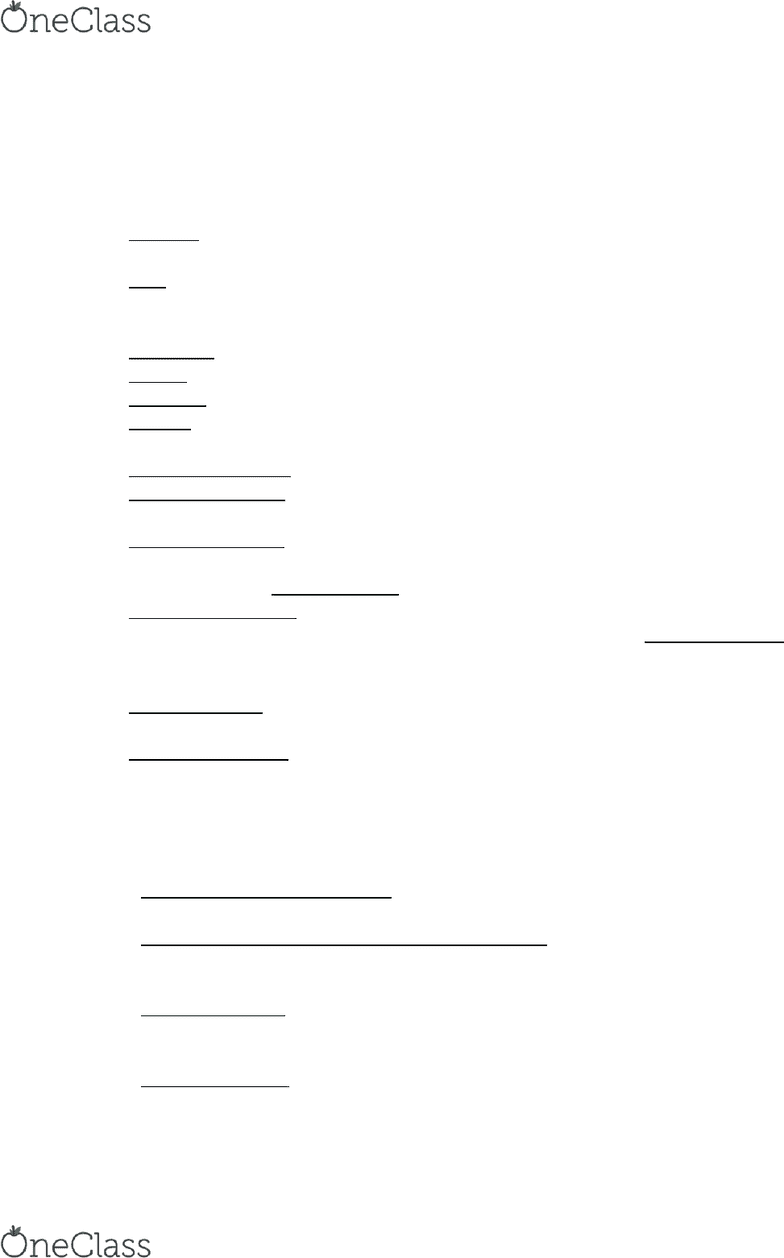# ST 210 Lecture 1: Ch.1a Notes

19 views3 pages
School
USA
Department
Statistics
Course
ST 210
ProfessorIntroduction to the Practice of Statistics
Vocabulary
Statistics - the science of collecting, organizing, summarizing and analyzing information to
draw conclusions
Data - a fact or proposition used to draw a conclusion or make a decision; list of observed
values for a variable
o Data describes characteristics and can be quantitative or qualitative
Population - the entire group to be studied
Sample - a subset of the population being studied
Individual - a person or object that is a member of population being studied
Statistic - a numerical summary of a sample
Parameter - a numerical summary of a population
Descriptive Statistics - describes data through numerical summaries, tables, and graphs
Inferential Statistics - take results from a sample, extend it to the population and measure
the reliability of the result
Qualitative Variable - or categorical, variables allow for classification of individuals based
on some attribute or characteristic (Gender, Zip Code) the corresponding data that is
collected is called Qualitative Data
Quantitative Variable - provide numerical measures of individuals (Temperature, days a
student studies) the corresponding data that is collected is called Quantitative Data
o The values of quantitative variables can be added or subtracted and provide
meaningful results
Discrete Variable - quantitative variable that has either a finite number of possible values
or a countable number of possible values
Continuous Variable - quantitative variable that has an infinite number of possible values
that are not countable
The Process of Statistics
Step 1: Identify the Research Objective
o The researchers need to determine detailed questions to be answered
Step 2: Collect the Data Needed to Answer the Question(s)
o Data collection is typically done on a sample of a population and choosing the
sample correctly is very important
Step 3: Describe the Data
o Descriptive statistics allow the researcher to obtain an overview of the data
collected
Step 4: Perform Inference
o Using different statistical techniques allow the results from the sample to be applied
to an entire population reliably
find more resources at oneclass.com
find more resources at oneclass.com
Unlock document

This preview shows page 1 of the document.
Unlock all 3 pages and 3 million more documents.